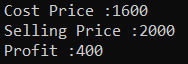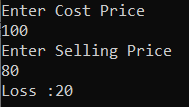# Profit and Loss Program in C#

## Profit and Loss Program in C# with Examples

In this article, I am going to discuss how to Calculate Profit and Loss in C# with Examples. Please read our previous article, where we discussed Fahrenheit to Celsius in C#.

Profit and Loss in C#

1. Cost Price (C.P.): The price at which the product is purchased, is called its cost price,
2. Selling Price (S.P.): The price at which the product is sold, is called its selling price,
3. Profit: If the Selling Price is greater than the Cost Price, the seller is said to have a profit or gain.
4. Loss: If the Selling Price is less than the Cost Price, the seller is said to have incurred a loss.
###### Formula.
1. Gain =(S.P.) – (C.P.)
2. Loss = (C.P.) – (S.P.)
3. Gain % = (Gain× 100÷C.P)
4. Loss % = (Loss× 100÷C.P) \
5. S.P = (100+Gain%× C.P ÷ 100)
6. S.P = (100-Loss% × C.P ÷ 100)
7. C.P = (100 × S.P ÷ 100 + Gain%)
8. C.P= (100 × S.P ÷ 100 – Loss%).
9. If the product is sold at a gain of say, 35%, then S.P. 135% of C.P.
10. If the product is sold at a loss of say, 35%, then S.P. = 65% of C.P.
11. When a person sells two similar items, one at a gain of say, x%, and the other At a loss of x%, then the seller always incurs a loss given by: Loss% = (Common Loss and Gain% ÷ 10) ^2 = (x÷10) ^2.

Where C.P is cost price, S.P is selling price, P is profit, and L is loss

###### Example:

A man buys one pen for Rs. 27.50 and sells it for Rs. 28.60. Find his gain.
Solution
C.P. = Rs. 27.50,
S.P. = Rs. 28.60.
So, profit = Rs. (28.60 – 27.50) = Rs. 1.10.

##### Logic to Calculate Profit and Loss in C#

To find the Profit and Loss simply we need to follow the below steps.

1. First, we will create the functions for Profit and Loss.
2. Then we will assign the value of cost price and selling price.
3. After that we have to follow the following steps: Check if cost price > selling price, then it is a loss find the difference and return the result. Check if selling price > cost price, then it is a profit find the difference and return the result. And if selling price == cost price, then there is no profit nor a loss.
4. After all the steps our result will print as output.
##### Example: Profit and Loss Program in C#.

The following C# Program will calculate the Profit and Loss.

```using System;
class ProfitAndLoss
{
// Function to calculate Profit
static double Profit(double costPrice, double sellingPrice)
{
double profit = (sellingPrice - costPrice);
return profit;
}
// Function to calculate Loss
static double Loss(double costPrice, double sellingPrice)
{
double Loss = (costPrice - sellingPrice);
return Loss;
}
public static void Main()
{
double costPrice = 1600, sellingPrice = 2000;
Console.WriteLine(" Cost Price :" + costPrice);
Console.WriteLine(" Selling Price :" + sellingPrice);
if (sellingPrice == costPrice)
Console.Write("No profit nor Loss");
else if (sellingPrice > costPrice)
{
Console.WriteLine(" Profit :" + Profit(costPrice, sellingPrice));
}
else
{
Console.WriteLine(" Loss :" + Loss(costPrice, sellingPrice));
}
}
}
```
###### Output:##### Example: Taking input from the user

In the following C# program, we will take the Cost Price and Selling Price from the user and then calculate the loss and profit and print it on the console.

```using System;
class ProfitAndLoss
{
// Function to calculate Profit
static double Profit(double costPrice, double sellingPrice)
{
double profit = (sellingPrice - costPrice);
return profit;
}
// Function to calculate Loss
static double Loss(double costPrice, double sellingPrice)
{
double Loss = (costPrice - sellingPrice);
return Loss;
}
public static void Main()
{
Console.WriteLine("Enter Cost Price ");
Console.WriteLine("Enter Selling Price ");
if (sellingPrice == costPrice)
Console.Write("No profit nor Loss");
else if (sellingPrice > costPrice)
{
Console.WriteLine("Profit :" + Profit(costPrice, sellingPrice));
}
else
{
Console.WriteLine("Loss :" + Loss(costPrice, sellingPrice));
}